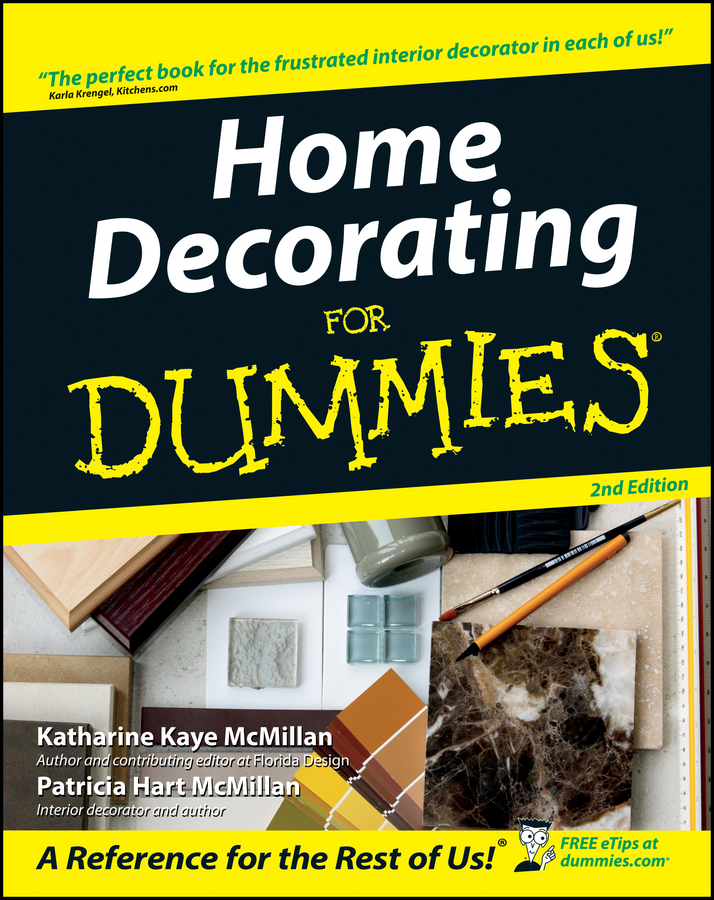##### Home Decorating For DummiesBefore you begin painting your home's interior walls, ceilings, woodwork, doors, or windows, you need to estimate the amount of paint you'll use. Estimates require specific calculations for each surface you want to paint.

To estimate the amount of paint you need in order to cover the walls of a room, add together the length of all the walls and then multiply the number by the height of the room, from floor to ceiling. The number you get is the room's square footage. Is that math class coming back to you now?

## How to determine square footage

Now you have to determine how much of that square footage is paintable surface area. Because you use a different paint on the doors and windows, subtract those areas from the room total. No sweat, just subtract 20 square feet for each door and 15 square feet for each average-sized window in the room. You end up with a number that is close to the actual wall area you have to cover with paint.

In general, you can expect 1 gallon of paint to cover about 350 square feet. You need slightly more than a gallon if the walls are unpainted drywall, which absorbs more of the paint. You also need to consider whether to paint more than one coat. If you're painting walls that are unfinished, heavily patched, or dark in color, plan on applying two coats of paint.

When painting a dark color, pros often add a color tint to the white primer. Tints for both latex or alkyd paints are available at most paint stores. For best results, choose a tint shade that's closest to the top coat color.

Now for the clincher of the math problem. Divide the paintable wall area by 350 (the square-foot coverage in each gallon can) to find the number of gallons of paint you need for the walls. You can round uneven numbers; if the remainder is less than .5, order a couple of quarts of wall paint to go with the gallons; if the remainder is more than .5, order an extra gallon. Of course, buying in bulk is usually more economical, so you may discover that 3 quarts of paint cost as much as a gallon.

## Examples of calculations

The following examples walk you through the calculations for determining how much paint you need for a 14-x-20-foot room that's 8 feet tall and has two doors and two windows.

### Ceiling paint estimator

Use the following formula to estimate the amount of ceiling paint you need. Double the result if the ceiling requires two coats.

1. Multiply the length of the ceiling times its width to find its area.

14 × 20 = 280 square feet

2. Divide that number by 350 (the estimated square feet covered per gallon) to figure out how many gallons of paint you need.

280 ÷ 350 = .8

For this example, you want to buy 1 gallon of ceiling paint for a single coat.

### Wall paint estimator

Use the following formula to estimate the amount of wall paint you need. Double the result if the walls require two coats.

1. Add together the length of each wall.

14 + 20 + 14 + 20 = 68 feet

2. Multiply the sum by the wall height, to find the total wall area.

68 × 8 = 544 square feet

3. Subtract 20 square feet for each door (20 × 2 = 40) and 15 square feet for each window (15 × 2 = 30) to find the actual amount of wall area you're painting.

544 – 70 = 474 square feet

4. Divide this figure by the paint coverage (350 square feet per gallon), and the result is the number of gallons to purchase.

474 ÷ 350 = 1.4

For this example, you want to buy 1 gallon and 2 quarts of paint for a single coat.

### Woodwork paint estimator

Measure the length of the trim in feet, and multiply that number by 1/2 foot (.5), as a rough size for the width of the trim. Include all the trim around doors and windows, at baseboards, along the ceiling, and for any built-in furniture.

As an example, imagine that you have ceiling molding running around a room that is 14 feet wide and 20 feet long.

1. Determine the total length of molding around the room by adding together the length of all the walls that the molding covers.

Round the numbers off to the nearest foot.

14 + 20 + 14 + 20 = 68 feet

2. Multiply the sum by .5 for an estimated width of the molding.

68 × .5 = 34 square feet

3. Divide this number by 350 to estimate the gallons of paint required to cover the molding.

34 ÷ 350 = .09

The result in this example is much less than a quart, but you may paint other woodwork in the room the same color, so buying a full quart may not be terribly wasteful.

### Door and window estimator

Use the same figure for estimating door coverage as you use in your wall-area calculations — 20 square feet = one door. Multiply the number of doors by 20, doubling the answer if you plan to paint both sides. Wall paint estimates allow for 15 square feet for each window. Use about half that window area to figure trim and inside sash — the glass isn't important to the calculation.

For the room in this example:

1. Multiply the number of doors by 20.

2 × 20 = 40 square feet

2. Multiply the number of windows by 7.5.

2 × 7.5 = 15 square feet

40 + 15 = 55

4. Divide the result by 350 (the estimated square feet covered per gallon).

54 ÷ 350 = .16

Often, you end up needing to buy only a quart of paint, which goes a long way on doors and window trim.